# linear transformation

Also found in: Dictionary, Acronyms, Wikipedia.

## linear transformation

[′lin·ē·ər ‚tranz·fər′mā·shən]
(mathematics)
A function T defined in a vector space E and having its values in another vector space over the same field, such that if ƒ and g are vectors in E, and c is a scalar, then T (ƒ + g) = T ƒ + Tg and T (c ƒ) = c (T ƒ). Also known as homogeneous transformation; linear function; linear operator.
McGraw-Hill Dictionary of Scientific & Technical Terms, 6E, Copyright © 2003 by The McGraw-Hill Companies, Inc.
The following article is from The Great Soviet Encyclopedia (1979). It might be outdated or ideologically biased.

## Linear Transformation

A linear transformation of the variables x1, x2, ..., xn is the replacement of these variables by new variables x′, x2,..., xn in terms of which the initial variables are expressed linearly, that is, by expressions of the form

x1 = a11x1 + a12x2 + ⋯ + annxn + b1

x2 = a21x1′ + a22x2′ + ⋯ + a2nxn′ + b2

. . . . . . . . . . . . . . . . . . . . . . . . . . . . . . . . . . . . .

xn = an1x1′ + an2x2′ + ⋯ + annxn′ + bn

Here aij and bi, for i, j = 1, 2,..., n, are arbitrary numerical coefficients. If b1, b2, ..., bn are all equal to zero, then the linear transformation of the variables is homogeneous.

As a simple example of a linear transformation of variables we can take the formulas for the transformation of rectangular coordinates in the plane

x = x′ cos α - y′ sin α + a

y = x′ sin α + y′ cos α + b

If determinant D = ǀaijǀ, formed by the multipliers of the variables is not equal to zero, then the new variables x1, x2,..., xn can also be linearly expressed in terms of the old variables. Thus, in our example,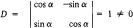and

x′ = x cos α + y sin α + a1

y′ = —x sin α + y cos α + b1

where a1 = —a cos αb sin α and b2 = a sin αb cos α. Other examples of linear transformations of variables are transformations of affine and (homogeneous) projective coordinates and the substitutions of variables involved in the reduction of quadratic forms.

A linear transformation of vectors (or a linear transformation on a vector space) is a rule that associates to a vector x in n-dimensional space a vector x′ whose coordinates are linear and homogeneous functions of the coordinates of x:

x1′ = a11x1 + a12x2 + ⋯ + a1nxn

x2′ = a21x1 + a22x2 + ⋯ + a2nxn

. . . . . . . . . . . . . . . . . . . . . . . . . . . . . . .

xn′ = an1x1 + an2x2 + ⋯ + annxn

or, briefly,

x′ = Ax

For example, projection to a coordinate plane, say the plane xOy, is a linear transformation of three-dimensional vector space. This transformation associates to each vector a with coordinates x, y, z a vector b, whose coordinates x′, y′, z′ are expressed in terms of x, y, z by x′ = x, y′ = y, and z′ = 0. An example of a linear transformation of the plane is its rotation through an angle α about the origin. The matrix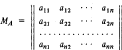formed by the coefficients of a linear transformation A, is the matrix of A. The matrices of the linear transformations of projection and rotation given above are, respectively,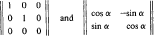A linear transformation on a vector space can be defined (as is generally done) without using a coordinate system. Thus, a correspondence x → y = Ax is a linear transformation if A(x + y) = Ax + Ay and A(αx) = αA(x) for any vectors x and y and for any number α. In different coordinate systems, different matrices (and, consequently, different formulas for the transformation of coordinates) correspond to the same linear transformation.

Linear transformations include, in particular, the zero transformation O that sends all vectors to the zero vector 0 (Ox = 0) and the identity transformation E that leaves all vectors invariant (Ex = x). In any coordinate system, these linear transformations are represented by the zero and identity matrix, respectively.

There are natural definitions of addition and multiplication of linear transformations on a vector space. Thus, the sum of two linear transformations A and B is the linear transformation C, which sends any vector x to the vector Cx = Ax + Bx, and the product of the linear transformations A and B is the result of their successive application: C = AB if Cx = A(Bx).

By virtue of these definitions, the set of all linear transformations of a vector space forms a ring. The matrix of a sum (product) of linear transformations is equal to the sum (product) of the matrices of the linear transformations. Since the product of linear transformations, like that of matrices, is not commutative, the order of the transformations in a product is important. A linear transformation can be multiplied by numbers. If the linear transformation A sends the vector x to the vector y = Ax, then αA sends x to αy. We illustrate these definitions by the following examples.

(1) If A and B denote the operation of projection to the Ox and Oy axes in three-dimensional space, then A + B will be the projection to the xOy plane, and AB = 0.

(2) If A and B are rotations of a plane about the origin through angles Φ and ψ, respectively, then AB is the rotation through the angle of Φ + ψ.

(3) The product of the identity transformation E and the number α is a dilation with ratio α.

The linear transformation B is the inverse of the linear transformation A (and is denoted by A-1) if BA = E (or AB = E). If A sends the vector x to the vector y, then A-1 sends y back to x. A linear transformation that possesses an inverse is called nonsingular. Such linear transformations are characterized by the fact that the determinants of their matrices are never equal to zero.

Certain classes of linear transformations deserve particular mention. Orthogonal (or unitary in complex spaces) linear transformations are a generalization of rotations of two-dimensional and three-dimensional Euclidean spaces. Orthogonal linear transformations do not alter the lengths of vectors (and, consequently, the angles between them). The matrices of these linear transformations relative to an orthonormal coordinate system are also called orthogonal (unitary). The product of an orthogonal matrix and its transpose is the identity matrix: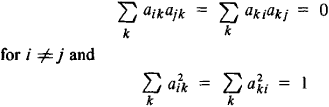In a complex space,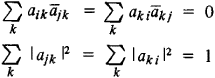A symmetric (in a complex space, Hermitian, or self-adjoint) linear transformation is a linear transformation whose matrix is symmetric, that is, aij = aji (or aij = āji). Symmetric linear transformations expand spaces by different coefficients in several mutually orthogonal directions. There is a close connection between the theory of quadratic forms (or Hermitian forms in a complex space) and symmetric linear transformations.

Our coordinate-free definition of a linear transformation on a vector space can be extended without any modifications to infinite-dimensional (in particular, function) spaces. Linear transformations on infinite-dimensional spaces are called linear operators.

### REFERENCES

Aleksandrov, P. S. Lektsii po analiticheskoi geometrii. Moscow, 1968.
Mal’tsev, A. I. Osnovy lineinoi algebry, 3rd ed. Moscow, 1970.
Efimov, N. V., and E. R. Rozendorn. Lineinaia algebra i mnogomernaia geometriia. Moscow, 1970.

## linear transformation

References in periodicals archive ?
L means [R.sup.16], i.e., the linear transformation layer, where R(b) = R([b.sub.15] [parallel] [b.sub.14] [parallel] [b.sub.13] *** [parallel] [b.sub.2] [parallel] [b.sub.1] [parallel] [b.sub.0]) = l([b.sub.15], ***, [b.sub.0]) [parallel] [b.sub.15] [parallel] [b.sub.14] [parallel] [b.sub.13] *** [parallel] [b.sub.2] [parallel] [b.sub.1] is a 16-byte LFSR.
In this research, a piecewise linear transformation function is used in order to develop an interpretable, logical, and fully invertible, where the inverse is also a function, nonlinear context adaptation process .
Information from sensory pathways, stored as digital information in digital cortices, is converted into experience information by a general linear transformation known as inhibition of lateral inhibition (ILI) in its sensory cortex, known to be the final information processing step on the pathways bringing information from the five senses to conscious awareness.
Then, the linear transformation algorithm is used to estimate the rough transmission map, , and finally, the Gaussian blur method is used to refine the rough transmittance function to obtain .
The Yld2000-2D anisotropic yield criterion  offers a symmetric yield surface, with two linear transformations onto the stress tensor, as follows
Then, we learn a linear transformation function that aligns the source subspace coordinate system to the target one.
Zhang, "Regression reformulations of LLE and LTSA with locally linear transformation," IEEE Transactions on Systems, Man, and Cybernetics, Part B: Cybernetics, vol.
And the weighted feature Gaussian basis kernel function is still a nonlinear model after using linear transformation. The conclusion can be proved by Theorem 3.
Wu, "Table-based linear transformation filters using OTA-C techniques", Electronics Letters, vol.
At first the principle of used robust methods will be explained (for this article only m-estimates), further their application to a linear transformation and the total processing procedure of the stage measurements.
Since linear transformation applied to Gaussian Random variables does not change its property , so we introduce such type of transformation as, Y = (X mx)/sx in order to convert this PDF into standard Gaussian PDF , whose mean is zero and variance equal to unity.
The following linear transformation is applied in each segment along its local coordinate axes:

Site: Follow: Share:
Open / Close